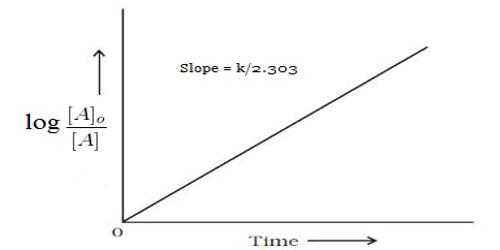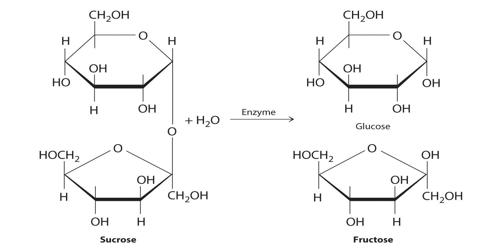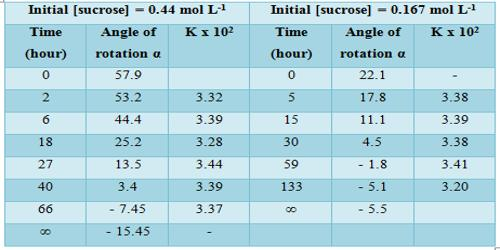# First-order Reaction: Hydrolysis of Sucrose to Glucose and Fructose

First-order Reaction: Hydrolysis of Sucrose to Glucose and Fructose catalyzed by acid

Hydrolysis of cane sugar (sucrose) to glucose and fructose catalyzed by acid follows first-order kinetics.

C12H22O11 (sucrose) + H2O + (H+) → C6H12O6 (glucose) + C6H12O6 (fructose) + (H+)

Since sucrose is optically active and dextrorotatory and an equimolar mixture of glucose and fructose is laevorotatory, the optical rotation of the plane of plane-polarized light will change during the progress of the above reaction. The glucose and fructose units are joined by an acetal oxygen bridge in the alpha-1 on the glucose and beta-2 on the fructose orientation. Consequently, the reaction rate can be conveniently followed by measuring the angle of rotation of the plane of polarization of plane-polarized light at suitable time intervals. The structure is simple to distinguish because it contains the six-member ring of glucose and the five-member ring of fructose.Let, α0 be the angle of rotation

α1 be the angle of rotation at time t.

αw be the angle of rotation at an infinite time (say after 72 hours)

Then, (α0 – αw) is proportional to initial concentration and (α0 – α1) is proportional to the concentration at time t.

Therefore [α0 – αw – (α0 – α1)] = (α1 – αw) is proportional to the concentration remaining unreacted at time t, i.e., (a – x).

These data when substituted in equation 12.22 yield,

k = 2.303/t log [(α0 – αw)/ (α1 – αw)]

Results shown in Table show the constancy of the values of k, so obtained over a wide concentration change. The specific reaction rate is also independent of the initial concentration of the sugar. In this case, the concentration of water (although it is a reactant) does not enter into the rate expression involving the two reactant species due to its presence in large excess in the reaction mixture. The hydrolysis of sucrose by boiling with a mineral acid, or by the enzyme invertase, produces a mixture of equal molecules of D-glucose and D-fructose. Such a reaction in which one reactant does not enter into the rate equation due to its presence in large excess is sometimes called pseudo-unimolecular reaction. It is to be noted that the acid in the reaction mixture acts as a catalyst. Hydrolysis can also be accelerated with acids, such as cream of tartar or lemon juice, both weak acids. Likewise, gastric acidity converts sucrose to glucose and fructose during digestion, the bond between them being an acetal bond which can be broken by an acid.

Sucrose is made from glucose and fructose units. The glucose and fructose units are joined by an acetal oxygen bridge in the alpha orientation. The construction is easy to recognize because it contains the six-member ring of glucose and the five-member ring of fructose.

Table: Hydrolysis of at 300 in 2.5 mol L-1 formic acidThe experimental data of this reaction can also be treated in a different manner by using the Guggenheim method of calculation. In this method, α0 and αw need not be known.